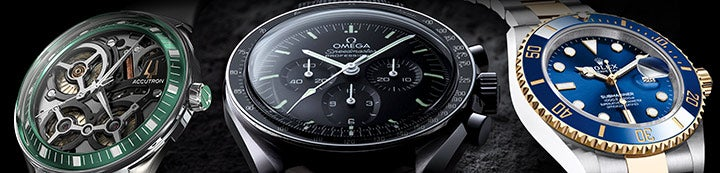1 - 1 of 1 Posts

aaron

·
Registered
Joined
·
3,680 Posts
Discussion Starter · ·
Hey Guys,

Saw this on another forum but it is cool.

Simply cut and paste the following in your address section and press go and watch the magic happen.

javascript:R= 0; x1=.1; y1=.05; x2=.25; y2=.24; x3=1.6; y3=.24; x4=300; y4=200; x5=300; y5=200; DI= document.images; DIL=DI.length; function A(){for(i=0; i<DIL; i++){DIS=DI[ i ].style; DIS.position= 'absolute' ; DIS.left=Math. sin(R*x1+ i*x2+x3)* x4+x5; DIS.top=Math. cos(R*y1+ i*y2+y3)* y4+y5}R++ }setInterval( 'A()',5); void(0)

The more photos in the section the better,even the sponsors names on this site take on a whole new look.

1 - 1 of 1 Posts